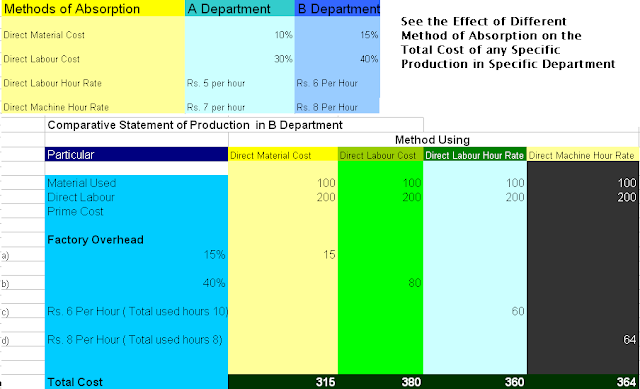222

# Why Accounting Education Important# Why Accounting Education Volunteer## Become Volunteer Teacher

After allocation and apportionment of overheads to their departments, we have to include it in the cost of production. The process of this is called absorption of overheads. For calculating the total cost of production, overhead is its main part. For including overhead in each unit of production, we have to study the methods of overhead absorption.

Following are the main methods of Overhead Absorption

1. Direct Material Cost Method

According to the material cost method, we calculate the rate of overhead on the basis of past actual direct material and past actual overheads. For example, past actual direct material's cost is Rs. 10,000 and past actual overhead cost is Rs. 2000. So, Overhead rate will be 20%. If we have obtained any specific order for producing any product, we will include the 20% cost of overhead. For example, we have produced one unit for our customer for this, we have used our Rs. 1000 material. Our overhead cost in it will be Rs. 1000 X 20% = Rs. 200. We this, we need not to go to deep study of calculating each overhead like electricity consumption in this one unit, depreciation for this one unit, salary cost for this one unit and other overhead cost for producing this one unit. Ok.

2. Direct Labour Cost Method

According to the Direct Labour Cost method, we calculate the rate of overhead on the basis of past actual cost of direct wages and past actual overheads

following is the formula

= Actual Overhead Cost / Direct Labour Cost X 100

For example, past actual direct wage's cost is Rs. 10,000 and past actual overhead cost is Rs. 2000. So, Overhead rate will be 20%. If we have obtained any specific order for producing any product, we will include the 20% cost of overhead. For example, we have produced one unit for our customer for this, we have paid Rs. 1000 to our laborers for their labour. Our overhead cost in it will be Rs. 1000 X 20% = Rs. 200.

3. Prime Cost Method

Under prime cost method, we calculate the actual or estimated prime cost in which direct material cost and direct labour cost will be added. We also calculate the budgeted Overhead Cost. After this, we calculate the rate of overhead. On this rate, we absorb our overhead cost on any new production.

Following is the formula of overhead rate

= Budgeted Overhead Expenses / Anticipated Prime Cost

4. Direct Labour Hour Method

Under this method of overhead absorption, we calculate the total direct labour hours by using our accounting information. We also see the total cost of overhead in these labour hours. After dividing this overhead cost with direct labour hours, we can easily calculate the rate of overhead. On this rate, we can absorb the estimated amount of overhead in any unit or units of production.

Following is the formula

= Overhead Cost / Direct labour Hours

For example, total direct labour hours are 10,000. Total overhead cost in these hours are Rs. 5,000. Our overhead rate is the 50% of labour hours in any production.

5.Machine Hour Rate Method

This is very good method of absorption of overhead cost in the industry where we do all works with the help of machines. We just have to calculate the machine hour rate and same rate will be the overhead rate and on this basis, we can absorb the estimated cost of overhead when we produce any unit of production. Following is the formula of calculating the machine hour rate

= Total Overhead Cost / Total Machine Hours

We have to apportion all the overhead cost on the basis of our machines.

a) Rent Expense

It is divided on the basis of area for each machine.

b) Lighting Expenses

It is divided on the basis of No. of Points for Each Machine

c) Supervision Cost

It is divided on the basis of time spent on each machine.

d) Insurance

It is divided on the basis of value of each machine.

e) Depreciation

It is divided on the basis of cost of each machine.

f) Power

It is divided on the basis of consumption by each machine.

g) Repair

It is divided on the basis of working life of each machine.

6. Rate Per Unit of Production Method

In this method, we calculated estimated overhead rate on the basis of units output and same is used for absorption of actual units of production.

=Budgeted Overhead Cost / Budgeted Units of Production

7. Sales Price Method

Under this method of absorption of overhead, we calculate the rate of overhead on the basis of sales of units and budgeted overhead cost.Same rate is used for absorption.

Formula

= Budgeted Overhead Expenses / Sales of Units of Production

This method is useful for absorbing sales, distribution, research, development, promotion and advertising expenses.Name

ltr
item
Accounting Education: Methods of Overhead Absorption